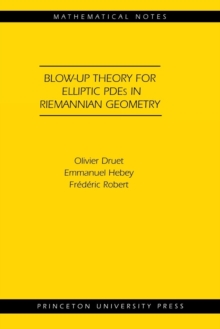Supporting your high street Find out how »
• My Account# Blow-up Theory for Elliptic PDEs in Riemannian Geometry (MN-45) Paperback / softback

## Part of the Mathematical Notes series

#### Description

Elliptic equations of critical Sobolev growth have been the target of investigation for decades because they have proved to be of great importance in analysis, geometry, and physics.

The equations studied here are of the well-known Yamabe type.

They involve Schrodinger operators on the left hand side and a critical nonlinearity on the right hand side.

A significant development in the study of such equations occurred in the 1980s.

It was discovered that the sequence splits into a solution of the limit equation--a finite sum of bubbles--and a rest that converges strongly to zero in the Sobolev space consisting of square integrable functions whose gradient is also square integrable.

This splitting is known as the integral theory for blow-up.

In this book, the authors develop the pointwise theory for blow-up.

They introduce new ideas and methods that lead to sharp pointwise estimates.

These estimates have important applications when dealing with sharp constant problems (a case where the energy is minimal) and compactness results (a case where the energy is arbitrarily large).

The authors carefully and thoroughly describe pointwise behavior when the energy is arbitrary. Intended to be as self-contained as possible, this accessible book will interest graduate students and researchers in a range of mathematical fields.

#### Information

• Format: Paperback / softback
• Pages: 224 pages
• Publisher: Princeton University Press
• Publication Date:
• Category: Differential calculus & equations
• ISBN: 9780691119533

#### Other Formats

£66.00

£49.29

on all orders

###### Pick up orders

from local bookshops

£73.95

£73.49

£79.20

£59.40

£74.00

£54.99

£104.00

£82.05

£62.00

£45.65

£115.00

£90.45